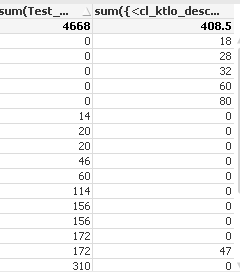# QlikView App Development

Discussion Board for collaboration related to QlikView App Development.

Announcements
Modernize Your QlikView Deployment webinar, Nov. 3rd. REGISTER
cancel
Showing results for
Did you mean:
HighlightedChampion III

## Re:Sum and exclude '"0"

hi all,

I have expression lik below

=num(

sum(Complexity)

/

(11111

sum({<desc={'Design - IT',' Design - RT'}>}hrs)

/8

)

,'##.##')i used same formualue in   straitght table  nummerator and denomenator.

am getting the sum of abve /sum of below exp.

My req is---   where  sum({<desc={'Design - IT',' Design - RT'}>}hrs)    this value is '0' shwn in chart  that corresponding values shud nt count and  remaing shud be count / total of sum({<desc={'Design - IT',' Design - RT'}>}hrs) .

example:  there is 47 value.. corresponig 172...   the result shud be   172/408.  is my result.

1 Solution

Accepted Solutions
HighlightedMVP & Luminary

Final expression is

=Sum(Aggr(If(sum({<cl_ktlo_desc={'Test - Design - SIT','Test - Design - RT'}>}cl_ktlo_hrs) > 0, sum(Test_Complexity), 0), Project, Period)) /

sum({<cl_ktlo_desc={'Test - Design - SIT','Test - Design - RT'}>}cl_ktlo_hrs)

87 Replies
HighlightedNot applicable

You Can write in If statement i.e if(sum({<desc={'Design - IT',' Design - RT'}>}hrs)>0,sum({<desc={'Design - IT',' Design - RT'}>}hrs))

HighlightedChampion

any sample app?

HighlightedMVP

This expression should be sufficient. The values divided by zero will be nulls:

=sum(Complexity) / sum({<desc = {'Design - IT', ' Design - RT'}>} TOTAL hrs)

NOTE the leading space in " Design - RT" - that is as per your posting but may be a typo. So it may be:

=sum(Complexity) / sum({<desc = {'Design - IT', 'Design - RT'}>} TOTAL hrs)

If you want zeroes instead of nulls:

=alt(sum(Complexity) / sum({<desc = {'Design - IT', 'Design - RT'}>} TOTAL hrs), 0)

Logic will get you from a to b. Imagination will take you everywhere. - A Einstein
HighlightedMaster

Hi Suresh,

Whats the text format in your hours field!

Is it just number or time format.

-Hirish

HirisH
“Aspire to Inspire before we Expire!”
HighlightedMVP & Luminary

Hi,

Try to add a If() like below

If(sum({<desc={'Design - IT',' Design - RT'}>}hrs) <> 0, Your expression)

Hope this helps you.

Regards,

Jagan.

HighlightedChampion III

hi,

hirish,

the format is..... 0.5   ,1,1.5,2,2.5  lik dat

HighlightedChampion III

i used but the values are chnging jagan...nt wrkng exacty

HighlightedChampion III

no jonathan.

As  u said the expesiion is Ok.

but i need only     this exp :  sum(Complexity)   values correponding to  second exp values where 0 is there.

i need to caluculate

there is 47 value.. corresponig 172...   the result shud be   172/408.  is my result.

172 value is  sum(cmp)  / 408  means    sum({<desc={'Design - IT',' Design - RT'}>}hrs)

HighlightedChampion III

chk below imagein abve image

where the values both sides  shud nt be calucate and          sum(comp)  value not equal to zero den only sum and divde with total sum    means sum(hrs)

ex: 14/56    sum(cmp)/Sum(hrs)      where exclude the  zero